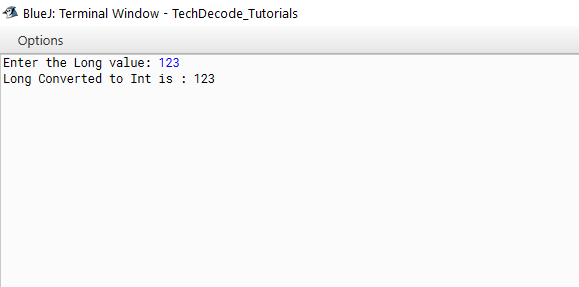# How to convert Long to Int in Java.

The primitive data type in Java is represented by the int keyword. It’s where variables are declared. It can also be used with methods that return values of the integer type. A 32-bit signed two’s complement integer can be stored in it.

The concept of an integer is familiar to most of us from our elementary school days. An integer is a number that doesn’t have a fractional component. In other words, an integer is comparable to a whole number, except it also has negative values. Thus, non-decimal numbers may both be positive and negative and It refers to a memory location (or locations) that is used to store non-decimal or non-fractional positive or negative numbers. The keyword ‘int’ signifies an integer type, which describes the memory needed to store the data. Additionally, this type has limitations on how it can be used. For example, fractions cannot be stored in integers. Now let us know a bit about Long data type as We are going to learn How to convert Long to Int in Java.

A 64-bit two’s complement integer is the long data type. The signed long has a value of – (2^63) at its lowest point and (2^63)-1 at its highest point. You can express an unsigned 64-bit long with a minimum value of 0 and a maximum value of (2^64)-1 with the long data type in Java SE 8 and later. When you need a larger range of values than an int can supply, use this data type. To allow arithmetic operations for unsigned longs, the Long class has methods like compareUnsigned(), divideUnsigned(), and so on

## What’s The Approach

We can do this program in 2 ways:-

• Import the “`java.util`” package.

• Create a `Scanner` class.

• Accept the input from the `user` .

• Convert the `Long` to `int` using the `typecasting`

• The Long can be converted to Int even by using the` intValue ()` method.

• Print the int value.

Also Read: – How to Convert Char To String in Java

### 1. Method

```/*
* TechDecode Tutorials
*
* How to Covert Long to Int
*
*/

import java.util.*;
class Long_to_Int
{
public static void main(String args[])
{
//Creating Scanner Class
Scanner sc=new Scanner(System.in);
//Accpeting users input
System.out.print("Enter the Long value: ");
long l =sc.nextLong();
//Converting to Int
int num = (int)l;
System.out.println("Long Converted to Int is : "+l);

}
}```

### 2.Method

```/*
* TechDecode Tutorials
*
* How to Covert Long to Int
*
*/

import java.util.*;
class Long_to_Int
{
public static void main(String args[])
{
//Creating Scanner Class
Scanner sc=new Scanner(System.in);
//Accpeting users input
System.out.print("Enter the Long value: ");
Long l = new Long(sc.nextLong());
//Converting to Int
int num = l.intValue();
System.out.println("Long Converted to Int is : "+l);

}
}```

Output: –